# RD Sharma Solutions for Class 6 Maths Chapter 8: Introduction to Algebra

RD Sharma Solutions for Class 6 Maths contains accurately designed exercise problems based on the understanding capacity of students. The solutions are created by subject matter experts according to the latest CBSE syllabus. It mainly helps students to solve problems based on the exam pattern and to clear doubts in an effective manner.

The solutions are prepared by keeping in mind concept based approach with accurate answering methods important from exam point of view. It contains well structured solutions for good conceptual knowledge among students. RD Sharma Solutions for Class 6 Maths Chapter 8 Introduction to Algebra are provided here.

## RD Sharma Solutions for Class 6 Maths Chapter 8: Introduction to Algebra Download PDF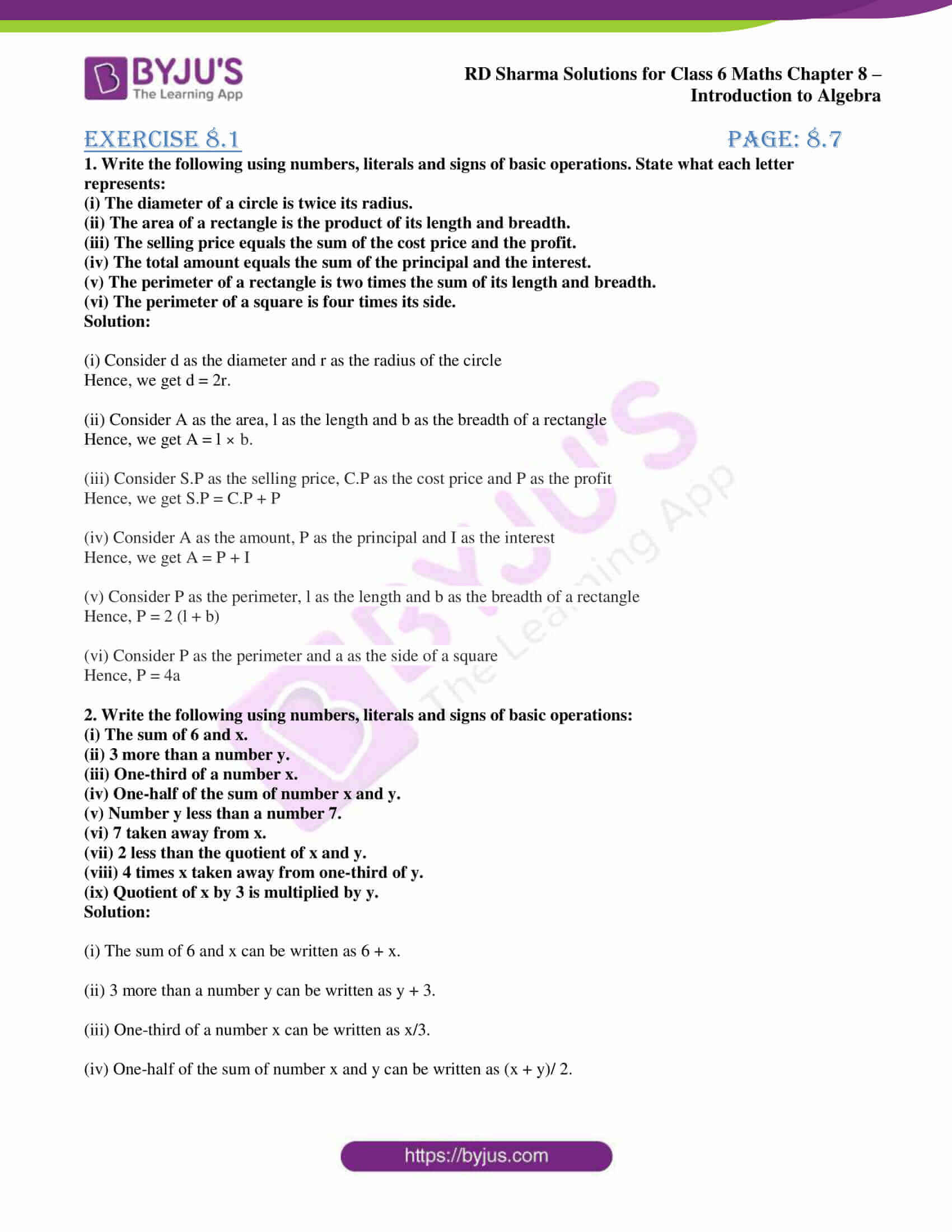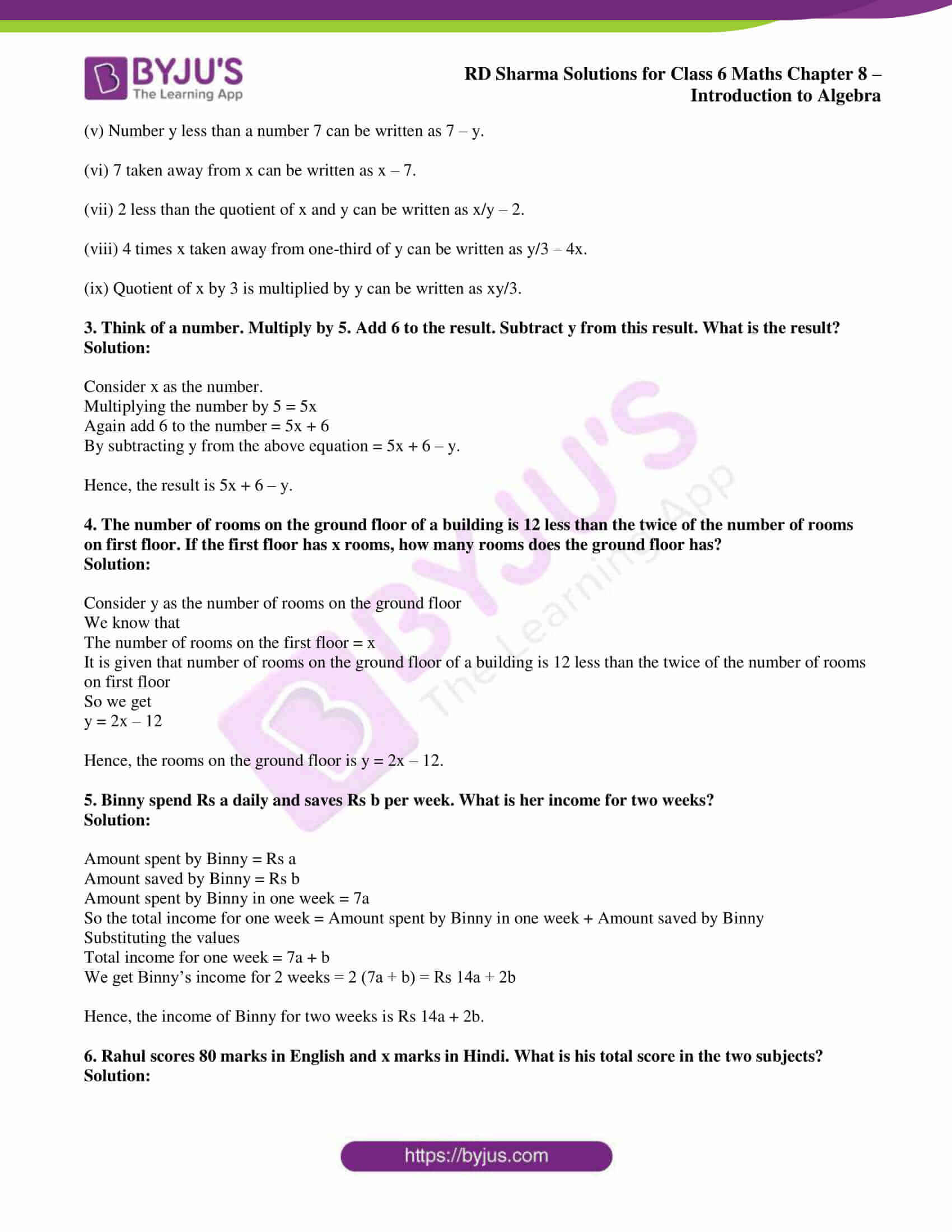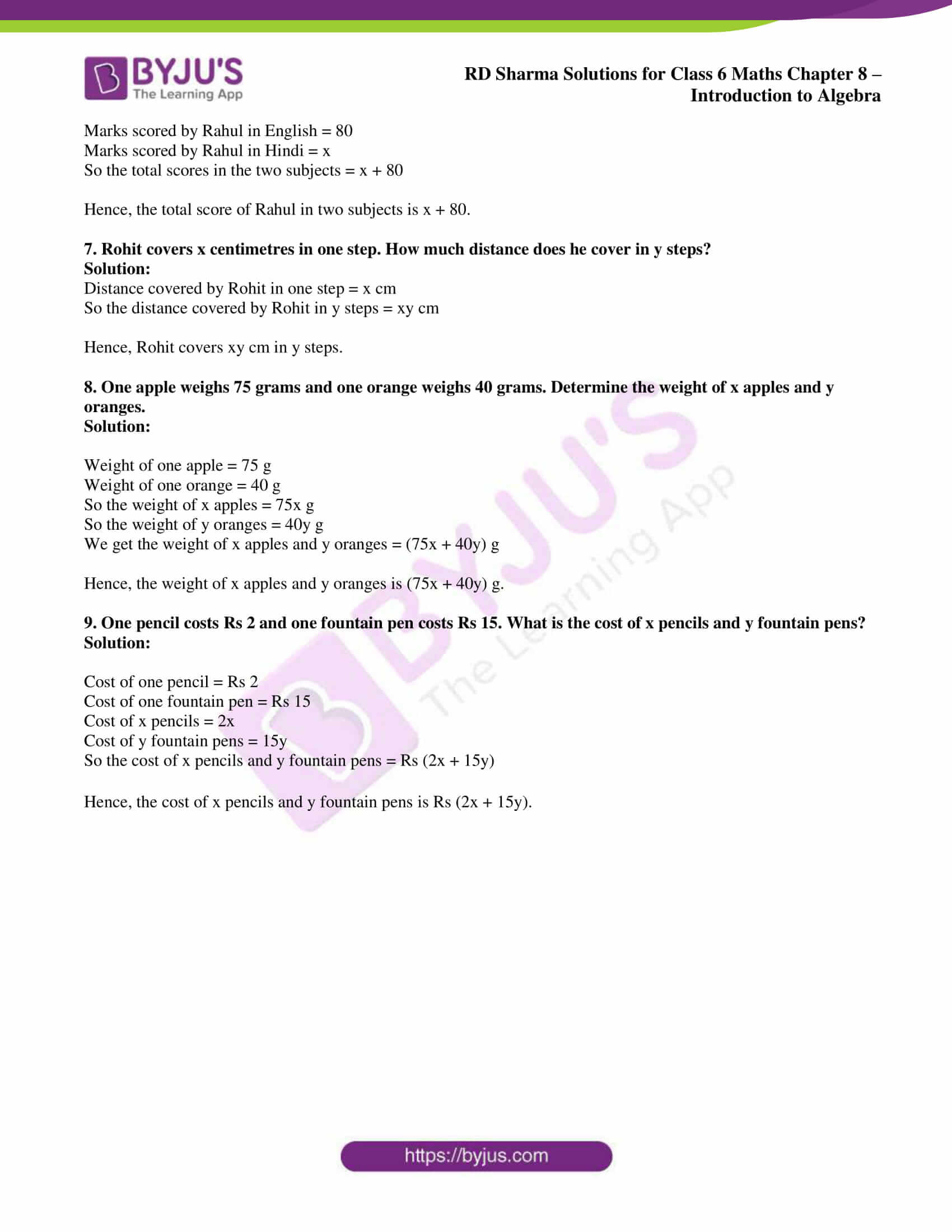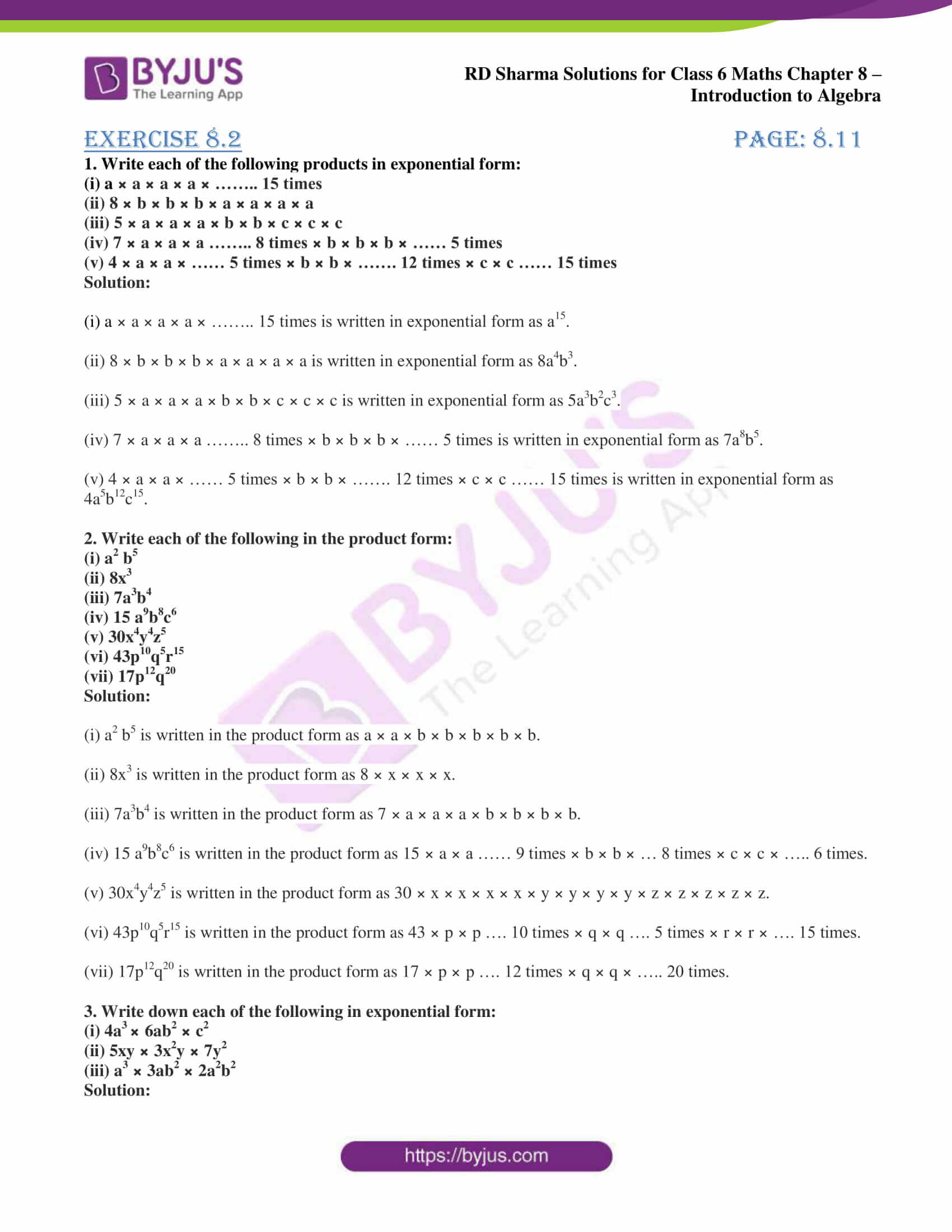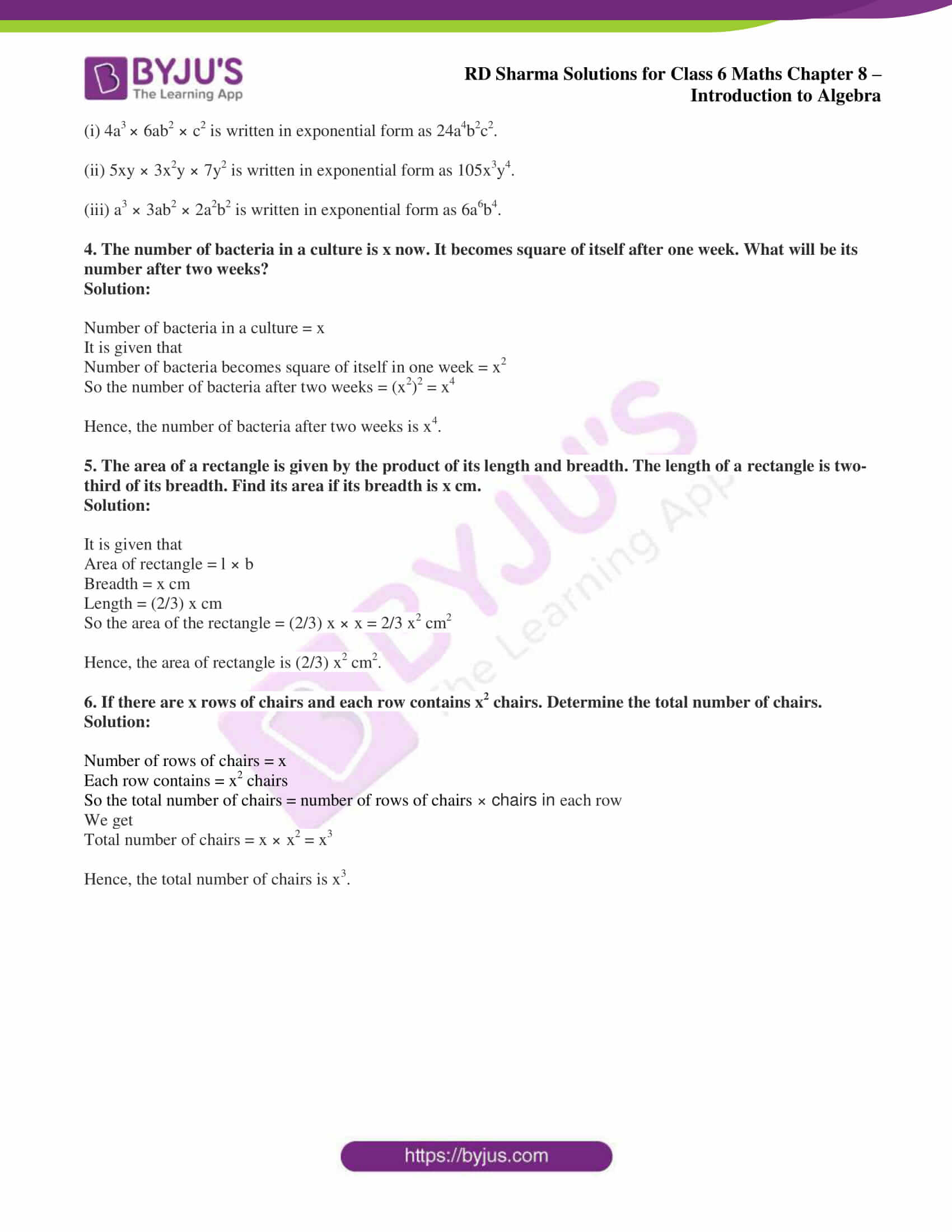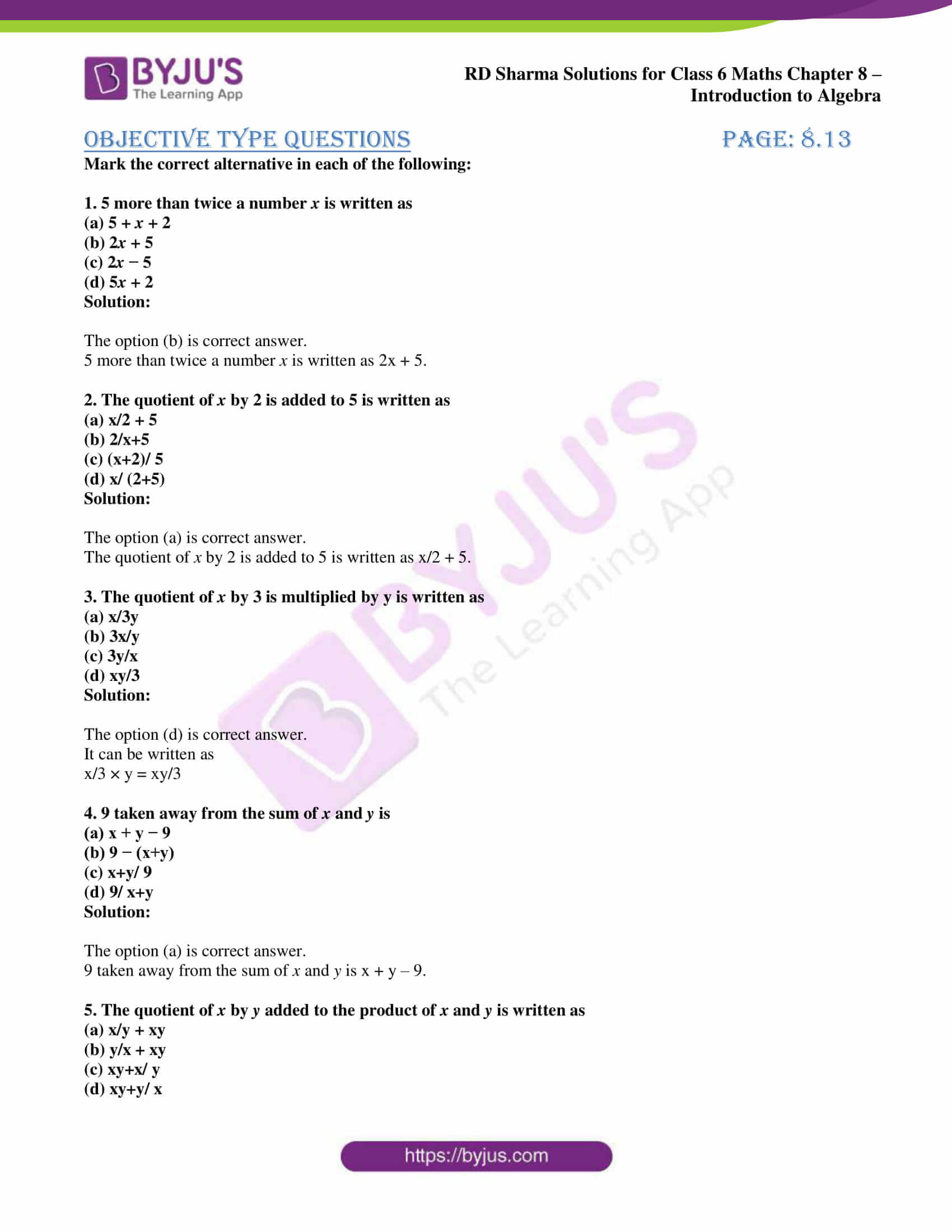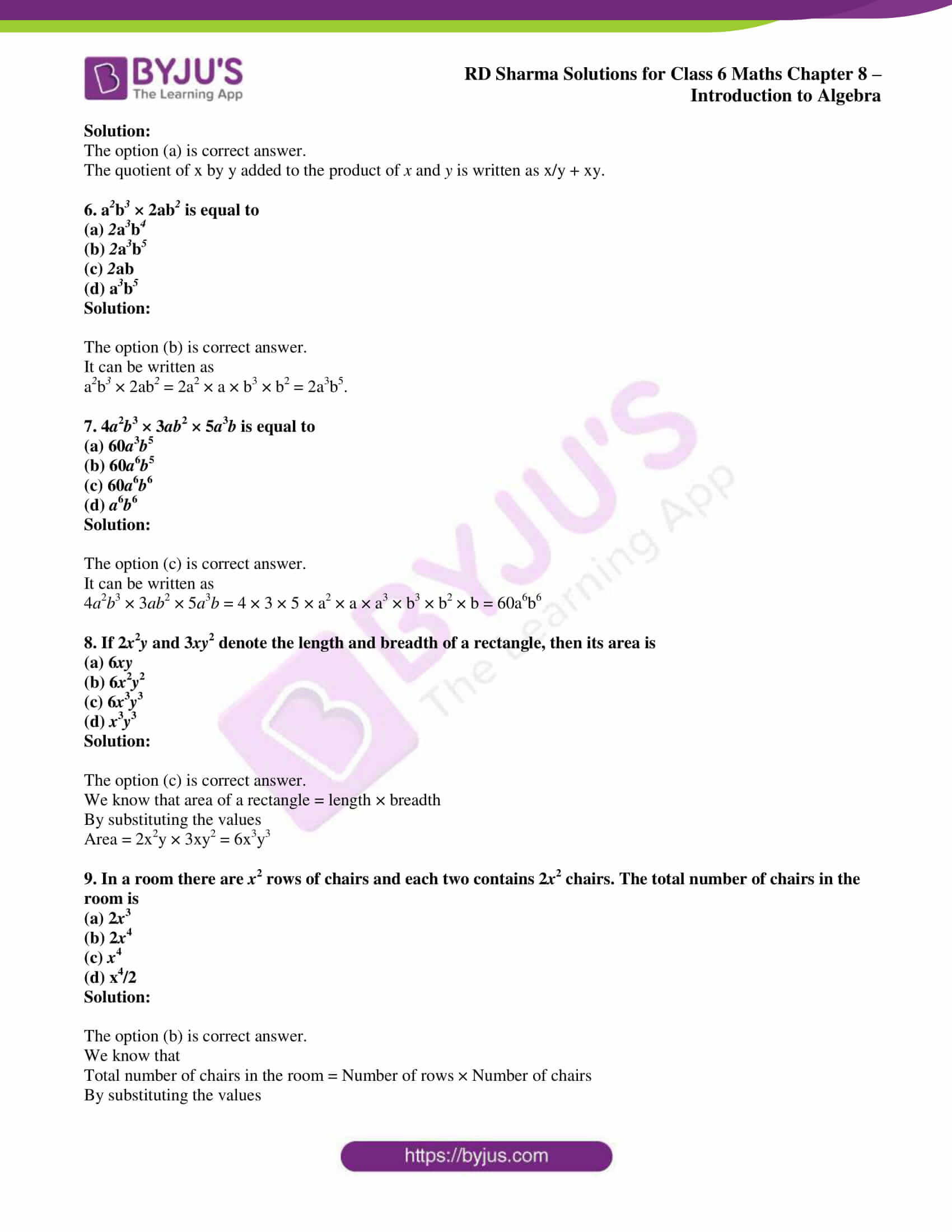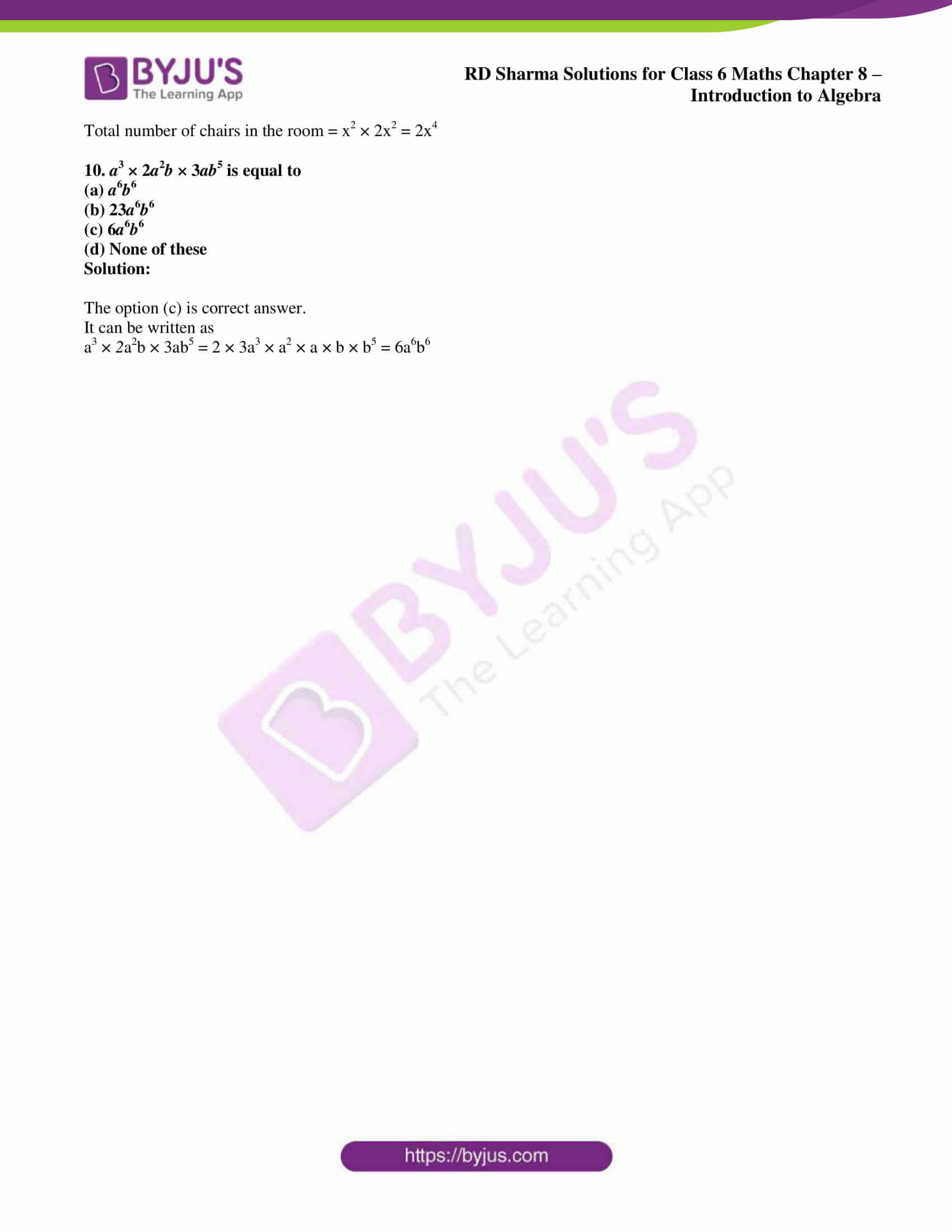## Access answers to Maths RD Sharma Solutions for Class 6 Chapter 8: Introduction to Algebra

### Exercise 8.1 page: 8.7

1. Write the following using numbers, literals and signs of basic operations. State what each letter represents:

(i) The diameter of a circle is twice its radius.

(ii) The area of a rectangle is the product of its length and breadth.

(iii) The selling price equals the sum of the cost price and the profit.

(iv) The total amount equals the sum of the principal and the interest.

(v) The perimeter of a rectangle is two times the sum of its length and breadth.

(vi) The perimeter of a square is four times its side.

Solution:

(i) Consider d as the diameter and r as the radius of the circle

Hence, we get d = 2r.

(ii) Consider A as the area, l as the length and b as the breadth of a rectangle

Hence, we get A = l × b.

(iii) Consider S.P as the selling price, C.P as the cost price and P as the profit

Hence, we get S.P = C.P + P

(iv) Consider A as the amount, P as the principal and I as the interest

Hence, we get A = P + I

(v) Consider P as the perimeter, l as the length and b as the breadth of a rectangle

Hence, P = 2 (l + b)

(vi) Consider P as the perimeter and a as the side of a square

Hence, P = 4a

2. Write the following using numbers, literals and signs of basic operations:

(i) The sum of 6 and x.

(ii) 3 more than a number y.

(iii) One-third of a number x.

(iv) One-half of the sum of number x and y.

(v) Number y less than a number 7.

(vi) 7 taken away from x.

(vii) 2 less than the quotient of x and y.

(viii) 4 times x taken away from one-third of y.

(ix) Quotient of x by 3 is multiplied by y.

Solution:

(i) The sum of 6 and x can be written as 6 + x.

(ii) 3 more than a number y can be written as y + 3.

(iii) One-third of a number x can be written as x/3.

(iv) One-half of the sum of number x and y can be written as (x + y)/ 2.

(v) Number y less than a number 7 can be written as 7 – y.

(vi) 7 taken away from x can be written as x – 7.

(vii) 2 less than the quotient of x and y can be written as x/y – 2.

(viii) 4 times x taken away from one-third of y can be written as y/3 – 4x.

(ix) Quotient of x by 3 is multiplied by y can be written as xy/3.

3. Think of a number. Multiply by 5. Add 6 to the result. Subtract y from this result. What is the result?

Solution:

Consider x as the number.

Multiplying the number by 5 = 5x

Again add 6 to the number = 5x + 6

By subtracting y from the above equation = 5x + 6 – y.

Hence, the result is 5x + 6 – y.

4. The number of rooms on the ground floor of a building is 12 less than the twice of the number of rooms on first floor. If the first floor has x rooms, how many rooms does the ground floor has?

Solution:

Consider y as the number of rooms on the ground floor

We know that

The number of rooms on the first floor = x

It is given that number of rooms on the ground floor of a building is 12 less than the twice of the number of rooms on first floor

So we get

y = 2x – 12

Hence, the rooms on the ground floor is y = 2x – 12.

5. Binny spend Rs a daily and saves Rs b per week. What is her income for two weeks?

Solution:

Amount spent by Binny = Rs a

Amount saved by Binny = Rs b

Amount spent by Binny in one week = 7a

So the total income for one week = Amount spent by Binny in one week + Amount saved by Binny

Substituting the values

Total income for one week = 7a + b

We get Binny’s income for 2 weeks = 2 (7a + b) = Rs 14a + 2b

Hence, the income of Binny for two weeks is Rs 14a + 2b.

6. Rahul scores 80 marks in English and x marks in Hindi. What is his total score in the two subjects?

Solution:

Marks scored by Rahul in English = 80

Marks scored by Rahul in Hindi = x

So the total scores in the two subjects = x + 80

Hence, the total score of Rahul in two subjects is x + 80.

7. Rohit covers x centimetres in one step. How much distance does he cover in y steps?

Solution:

Distance covered by Rohit in one step = x cm

So the distance covered by Rohit in y steps = xy cm

Hence, Rohit covers xy cm in y steps.

8. One apple weighs 75 grams and one orange weighs 40 grams. Determine the weight of x apples and y oranges.

Solution:

Weight of one apple = 75 g

Weight of one orange = 40 g

So the weight of x apples = 75x g

So the weight of y oranges = 40y g

We get the weight of x apples and y oranges = (75x + 40y) g

Hence, the weight of x apples and y oranges is (75x + 40y) g.

9. One pencil costs Rs 2 and one fountain pen costs Rs 15. What is the cost of x pencils and y fountain pens?

Solution:

Cost of one pencil = Rs 2

Cost of one fountain pen = Rs 15

Cost of x pencils = 2x

Cost of y fountain pens = 15y

So the cost of x pencils and y fountain pens = Rs (2x + 15y)

Hence, the cost of x pencils and y fountain pens is Rs (2x + 15y).

### Exercise 8.2 page: 8.11

1. Write each of the following products in exponential form:

(i) a × a × a × a × …….. 15 times

(ii) 8 × b × b × b × a × a × a × a

(iii) 5 × a × a × a × b × b × c × c × c

(iv) 7 × a × a × a …….. 8 times × b × b × b × …… 5 times

(v) 4 × a × a × …… 5 times × b × b × ……. 12 times × c × c …… 15 times

Solution:

(i) a × a × a × a × …….. 15 times is written in exponential form as a15.

(ii) 8 × b × b × b × a × a × a × a is written in exponential form as 8a4b3.

(iii) 5 × a × a × a × b × b × c × c × c is written in exponential form as 5a3b2c3.

(iv) 7 × a × a × a …….. 8 times × b × b × b × …… 5 times is written in exponential form as 7a8b5.

(v) 4 × a × a × …… 5 times × b × b × ……. 12 times × c × c …… 15 times is written in exponential form as 4a5b12c15.

2. Write each of the following in the product form:

(i) a2 b5

(ii) 8x3

(iii) 7a3b4

(iv) 15 a9b8c6

(v) 30x4y4z5

(vi) 43p10q5r15

(vii) 17p12q20

Solution:

(i) a2 b5 is written in the product form as a × a × b × b × b × b × b.

(ii) 8x3 is written in the product form as 8 × x × x × x.

(iii) 7a3b4 is written in the product form as 7 × a × a × a × b × b × b × b.

(iv) 15 a9b8c6 is written in the product form as 15 × a × a …… 9 times × b × b × … 8 times × c × c × ….. 6 times.

(v) 30x4y4z5 is written in the product form as 30 × x × x × x × x × y × y × y × y × z × z × z × z × z.

(vi) 43p10q5r15 is written in the product form as 43 × p × p …. 10 times × q × q …. 5 times × r × r × …. 15 times.

(vii) 17p12q20 is written in the product form as 17 × p × p …. 12 times × q × q × ….. 20 times.

3. Write down each of the following in exponential form:

(i) 4a3 × 6ab2 × c2

(ii) 5xy × 3x2y × 7y2

(iii) a3 × 3ab2 × 2a2b2

Solution:

(i) 4a3 × 6ab2 × c2 is written in exponential form as 24a4b2c2.

(ii) 5xy × 3x2y × 7y2 is written in exponential form as 105x3y4.

(iii) a3 × 3ab2 × 2a2b2 is written in exponential form as 6a6b4.

4. The number of bacteria in a culture is x now. It becomes square of itself after one week. What will be its number after two weeks?

Solution:

Number of bacteria in a culture = x

It is given that

Number of bacteria becomes square of itself in one week = x2

So the number of bacteria after two weeks = (x2)2 = x4

Hence, the number of bacteria after two weeks is x4.

5. The area of a rectangle is given by the product of its length and breadth. The length of a rectangle is two-third of its breadth. Find its area if its breadth is x cm.

Solution:

It is given that

Area of rectangle = l × b

Length = (2/3) x cm

So the area of the rectangle = (2/3) x × x = 2/3 x2 cm2

Hence, the area of rectangle is (2/3) x2 cm2.

6. If there are x rows of chairs and each row contains x2 chairs. Determine the total number of chairs.

Solution:

Number of rows of chairs = x

Each row contains = x2 chairs

So the total number of chairs = number of rows of chairs × chairs in each row

We get

Total number of chairs = x × x2 = x3

Hence, the total number of chairs is x3.

### Objective Type Questions PAGE: 8.13

Mark the correct alternative in each of the following:

1. 5 more than twice a number x is written as
(a) 5 + x + 2
(b) 2x + 5
(c) 2x − 5
(d) 5x + 2

Solution:

The option (b) is correct answer.

5 more than twice a number x is written as 2x + 5.

2. The quotient of x by 2 is added to 5 is written as
(a) x/2 + 5
(b) 2/x+5
(c) (x+2)/ 5
(d) x/ (2+5)

Solution:

The option (a) is correct answer.

The quotient of x by 2 is added to 5 is written as x/2 + 5.

3. The quotient of x by 3 is multiplied by y is written as
(a) x/3y
(b) 3x/y
(c) 3y/x
(d) xy/3

Solution:

The option (d) is correct answer.

It can be written as

x/3 × y = xy/3

4. 9 taken away from the sum of x and y is
(a) x + y − 9
(b) 9 − (x+y)
(c) x+y/ 9
(d) 9/ x+y

Solution:

The option (a) is correct answer.

9 taken away from the sum of x and y is x + y – 9.

5. The quotient of x by y added to the product of x and y is written as
(a) x/y + xy
(b) y/x + xy
(c) xy+x/ y
(d) xy+y/ x

Solution:

The option (a) is correct answer.

The quotient of x by y added to the product of x and y is written as x/y + xy.

6. a2b3 × 2ab2 is equal to
(a) 2a3b4
(b) 2a3b5
(c) 2ab
(d) a3b5

Solution:

The option (b) is correct answer.

It can be written as

a2b3 × 2ab2 = 2a2 × a × b3 × b2 = 2a3b5.

7. 4a2b3 × 3ab2 × 5a3b is equal to
(a) 60a3b5
(b) 60a6b5
(c) 60a6b6
(d) a6b6

Solution:

The option (c) is correct answer.

It can be written as

4a2b3 × 3ab2 × 5a3b = 4 × 3 × 5 × a2 × a × a3 × b3 × b2 × b = 60a6b6

8. If 2x2y and 3xy2 denote the length and breadth of a rectangle, then its area is
(a) 6xy
(b) 6x2y2
(c) 6x3y3
(d) x3y3

Solution:

The option (c) is correct answer.

We know that area of a rectangle = length × breadth

By substituting the values

Area = 2x2y × 3xy2 = 6x3y3

9. In a room there are x2 rows of chairs and each two contains 2x2 chairs. The total number of chairs in the room is
(a) 2x3
(b) 2x4
(c) x4
(d) x4/2

Solution:

The option (b) is correct answer.

We know that

Total number of chairs in the room = Number of rows × Number of chairs

By substituting the values

Total number of chairs in the room = x2 × 2x2 = 2x4

10. a3 × 2a2b × 3ab5 is equal to
(a) a6b6
(b) 23a6b6
(c) 6a6b6
(d) None of these

Solution:

The option (c) is correct answer.

It can be written as

a3 × 2a2b × 3ab5 = 2 × 3a3 × a2 × a × b × b5 = 6a6b6

### RD Sharma Solutions for Class 6 Maths Chapter 8: Introduction to Algebra

Chapter 8, Introduction to Algebra, has 2 exercises which explains the various operations on algebraic terms and the concept of variables and constants. The major concepts which are talked about in RD Sharma Solutions Chapter 8 are as listed below:

• Introduction
• Use of Letters to Denote Numbers
• Basic Operations on Literals and Numbers
• Powers of Literal Numbers
• Variables and Constants

### Chapter Brief of RD Sharma Solutions for Class 6 Maths Chapter 8 – Introduction to Algebra

The solutions are created by experts at BYJU’S with the aim of helping students perform well in the exam. The well designed solutions for each chapter help students improve conceptual knowledge and problem solving abilities. By solving the exercise wise problems, students self analyse the areas of weaknesses and work on them.

Some of the major applications of Algebra in day to day lives are Finance, long term investments, the lowest cost method of Financing, Sports, Technology, Business etc. By downloading the PDF of solutions, students gain a better knowledge about Algebra and methods used in solving exercise wise problems.